#### A PHP Error was encountered

Severity: Warning

Message: count(): Parameter must be an array or an object that implements Countable

Filename: controllers/formulas.php

Line Number: 37

Cos Formula Online - Tutorpace

# Cos Formula

## Online Tutoring Is The Easiest, Most Cost-Effective Way For Students To Get The Help They Need Whenever They Need It.

Cos formula is an effective tool in trigonometric functions.  Cos formula is based on right angle triangle. In right angle triangle with legs a, b and c and angle k is opposite to side a, the cos angle k is defined as length of adjacent side divide by length of hypotenuse.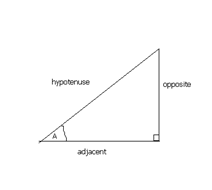In simple cos (A) = adjacent / hypotenuse
The following examples illustrates the use of cos formula
Example 1: If X = 16, Y = 30, and Z = 34, what is the cos of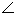A?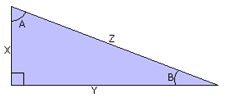Solution: In the right triangle, the cosine of A is the ratio of the side adjacent A to the hypotenuse.
=> Cos (A) = adjacent / hypotenuse
=> Cos (A) = X / Z
=> Cos (A) = 16 / 34 = 0.471
=> Therefore cos (A) = 0.471.

Example 2: Find the size of a0?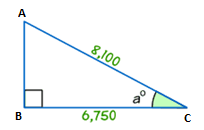Solution: From the figure we know
=> Length of adjacent side = 6750
=> Length of hypotenuse = 8100
=> Use cos formula: Cos (a) = adjacent / hypotenuse
=> So, cos (a) = 6750/8100 = 0.83333
=> Find the angle using calculator cos^-1(0.83333)
=> cos^-1(0.83333) = 33.6

Example 3: Find the length of x in the below figure?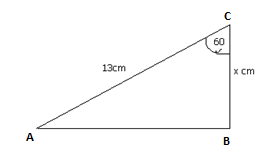Solution: In figure it is shown that the angle is 60 degrees.
=> The hypotenuse is given as 13cm and we need to find the adjacent side. This formula that connects angle, hypotenuse and adjacent side is cos formula.

=> We know that Cos (angle) = adjacent side / hypotenuse

=> Therefore, cos (60) = x / 13

=> We know that Cos (60) = ½ = 0.5

=> So, x = 13 × cos (60) = 13 x 0.5 = 6.5

=> Hence, the length of   x = 6.5cm